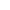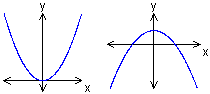Go to content
To plot the graph of a quadratic function you must first create a table of values. Then choose suitable axes that will allow all these values to be plotted. Plot the values, then draw a smooth curve through the points.## Summary/BackgroundThese curves are called quadratic curves. When you plot them on a graph they are shaped like a hill or a valley. They always have a vertical line of symmetry going down through the middle.You can get a better display of the maths by downloading special TeX fonts from jsMath. In the meantime, we will do the best we can with the fonts you have, but it may not be pretty and some equations may not be rendered correctly.

## Glossary

### axes

One of two straights line on a graph from which measurements are taken. One axis (the y axis) is vertical; the other (the x axis)is horizontal.

### function

A rule that connects one value in one set with one and only one value in another set.

### graph

A diagram showing a relationship between two variables.
The diagram shows a vertical y axis and a horizontal x axis.

### plot

To mark a point on a graph accurately by using its coordinates.

A mathematical expression of the 2nd degree; having a x² term but no higher one.

### smooth

having negligible friction

### union

The union of two sets A and B is the set containing all the elements of A and B.

Full Glossary List

## This question appears in the following syllabi:

SyllabusModuleSectionTopicExam Year
AQA GCSE (9-1) Foundation (UK)A: GraphsA12: Sketching Graphs of FunctionsGraphs of Quadratics-
CBSE IX (India)AlgebraPolynomialsConstant, linear, quadratic and cubic-
CIE IGCSE (9-1) Maths (0626 UK)2 Algebra and GraphsB2.10 Further GraphingGraphs of Quadratics-
Edexcel GCSE (9-1) Foundation (UK)A: GraphsA12: Sketching Graphs of FunctionsGraphs of Quadratics-